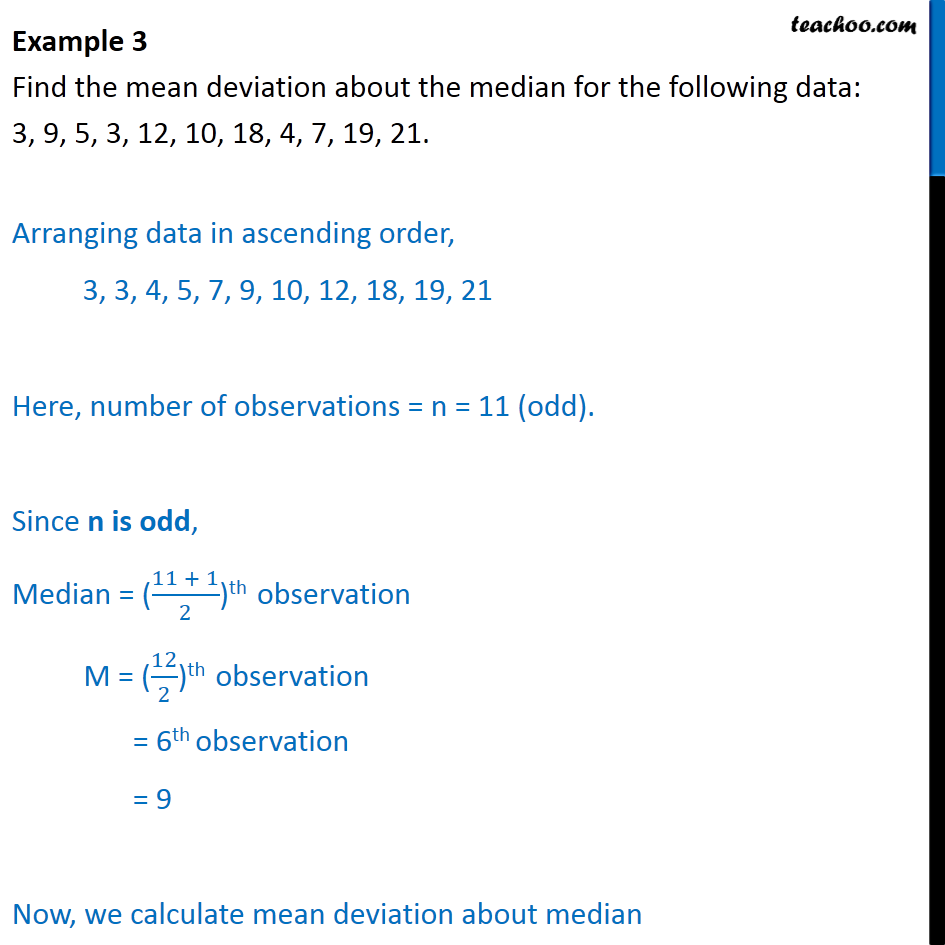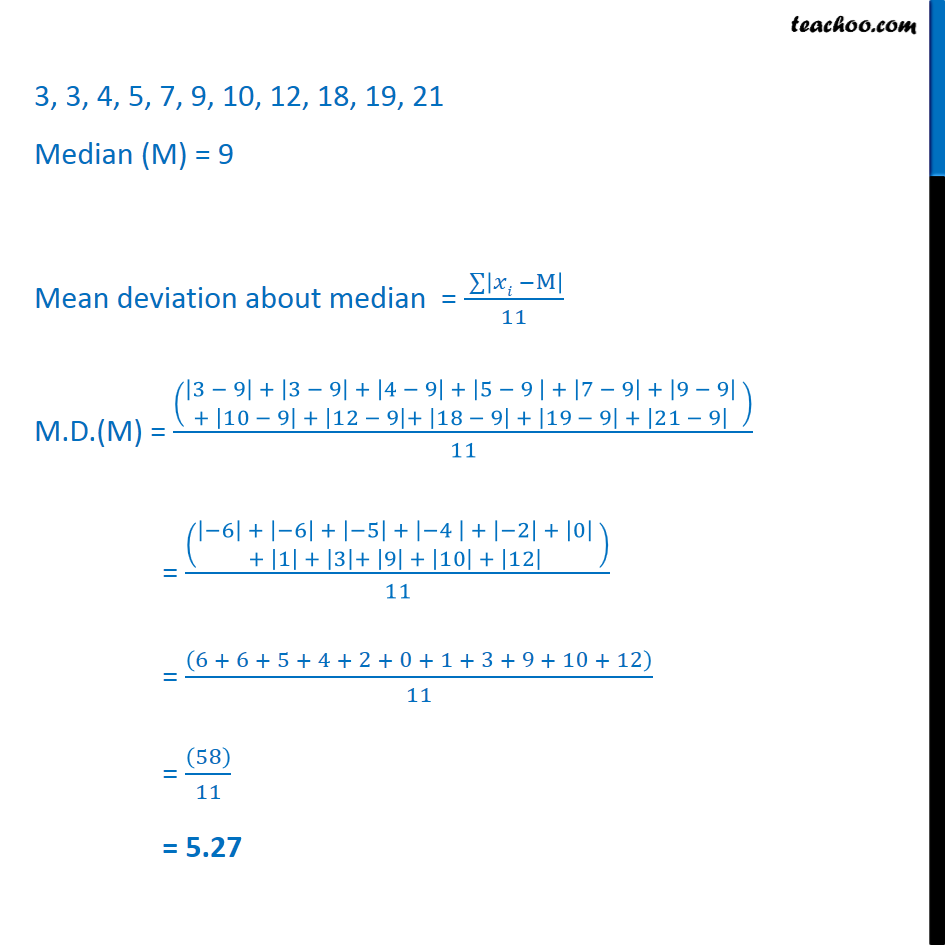Mean deviation about median - Ungrouped

Chapter 13 Class 11 Statistics
Concept wiseLearn in your speed, with individual attention - Teachoo Maths 1-on-1 Class

### Transcript

Example 3 Find the mean deviation about the median for the following data: 3, 9, 5, 3, 12, 10, 18, 4, 7, 19, 21. Arranging data in ascending order, 3, 3, 4, 5, 7, 9, 10, 12, 18, 19, 21 Here, number of observations = n = 11 (odd). Since n is odd, Median = ( 11 + 1﷮2﷯)th observation M = ( 12﷮2﷯)th observation = 6th observation = 9 Now, we calculate mean deviation about median 3, 3, 4, 5, 7, 9, 10, 12, 18, 19, 21 Median (M) = 9 Mean deviation about median = ﷮﷮ 𝑥𝑖 −M﷯﷯﷮11﷯ M.D.(M) = 3 − 9﷯ + 3 − 9﷯ + 4 − 9﷯ + 5 − 9 ﷯ + 7 − 9﷯ + 9 − 9﷯ ﷮+ 10 − 9﷯ + 12 − 9﷯+ 18 − 9﷯ + 19 − 9﷯ + 21 − 9﷯ ﷯﷯﷮11﷯ = −6﷯ + −6﷯ + −5﷯ + −4 ﷯ + −2﷯ + 0﷯ ﷮+ 1﷯ + 3﷯+ 9﷯ + 10﷯ + 12﷯ ﷯﷯﷮11﷯ = 6 + 6 + 5 + 4 + 2 + 0 + 1 + 3 + 9 + 10 + 12﷯﷮11﷯ = 58﷯﷮11﷯ = 5.27